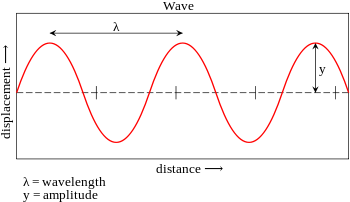# Representing a Wave

A wave is represented in terms of its wavelength, amplitude, frequency and time period.Amplitude

The maximum height of the wave.

Wavelength

The distance between adjacent troughs or adjacent crests, measured in unit of length such as meters and expressed by symbol λ (lambda). For longitudinal wave, it will be distance between two successive rarefactions or compressions.

Time Period

This defines the time it takes for one complete wave to pass a given point, measured in seconds (s).

Frequency

The number of complete waves that pass a point in one second, measured in Hertz (Hz).

Speed or velocity

Wave speed is defined as the distance travelled by a wave disturbance in one second and is measured in meters/second (ms-1). Speed is scalar quantity while velocity is a vector quantity.

### Relation Between Properties

Period is inversely related to the frequency. This means if the frequency is high, the period will be low.

T = 1/n

Velocity = frequency x wavelength

V = n x λ

The waves that produce a sense of sound for living beings are called sound waves or audible waves. Only those waves that have frequencies lying in the range of 16 Hz to 20,000 Hz are audible to human beings.

Sound waves with frequencies below 16 Hz are called infrasonic waves and those above 20 kHz are ultrasonic waves. Animals like bats are able to produce and sense waves beyond the range of human audibility and use it for seeing in the dark.